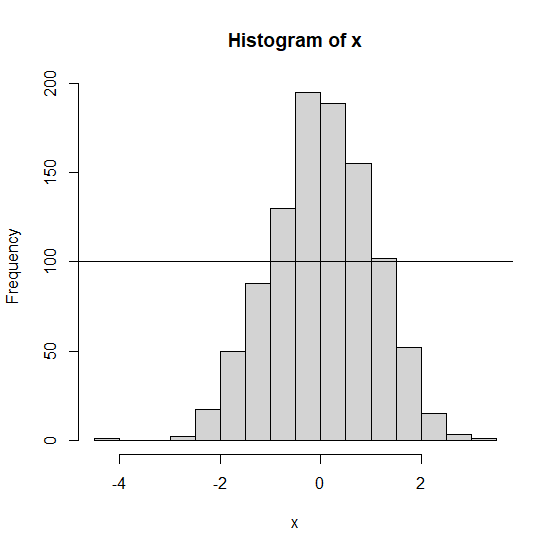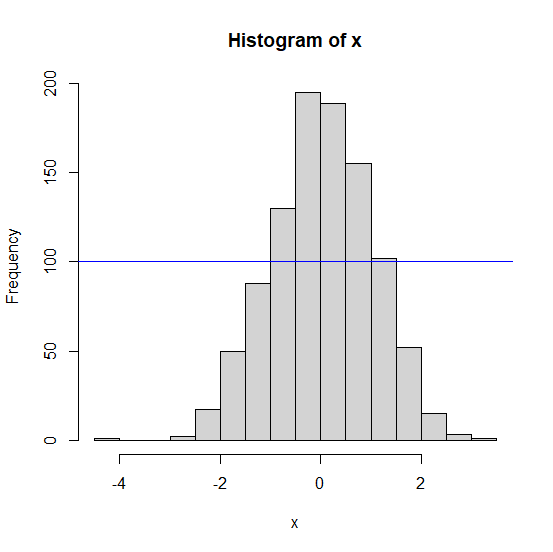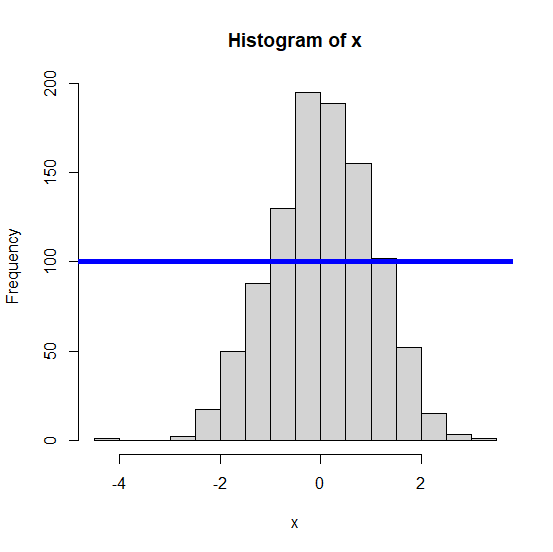# How to create a horizontal line in a histogram in base R?

A horizontal line in a histogram is not much useful but we might want to create it in some situations such as where we want to display a specific value on the Y-axis that helps us to differentiate in the density of frequencies above or below a certain value. To create a horizontal line in a histogram, we simply need to use abline function as shown in the below example

## Example

> x<-rnorm(1000)
> hist(x)
> abline(h=100)

## Output## Example

> abline(h=100,col="blue")

## Output## Example

> abline(h=100,col="blue",lwd=5)

## Output